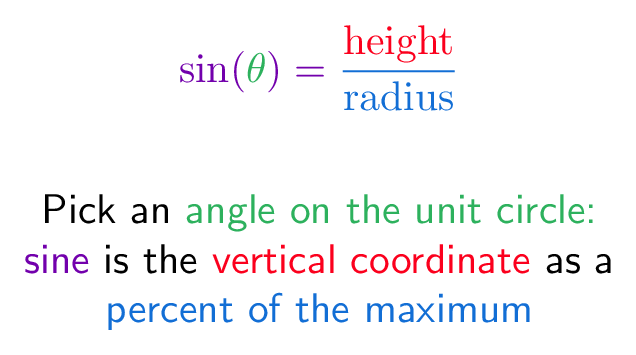# # Sine (Unit Circle)

## # Colorized Definition\newcommand{\sinefunction}{\color{c1}}
\newcommand{\thetacolor}{\color{c2}}
\newcommand{\opposite}{\color{c3}}
\newcommand{\hypotenuse}{\color{c4}}

$$\sinefunction \sin(\thetacolor{\theta} \sinefunction) = \hypotenuse \frac{\opposite \textrm{height}}{\textrm{radius}}$$

\plain Pick an \thetacolor angle on the unit circle:
\\
\sinefunction sine \plain is the \opposite vertical coordinate
\plain as a
\\
\hypotenuse percent of the maximum